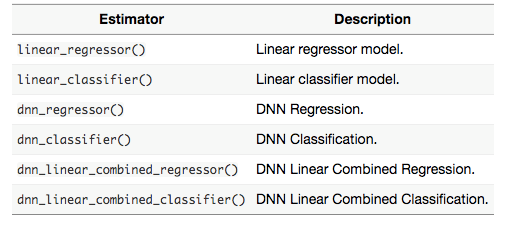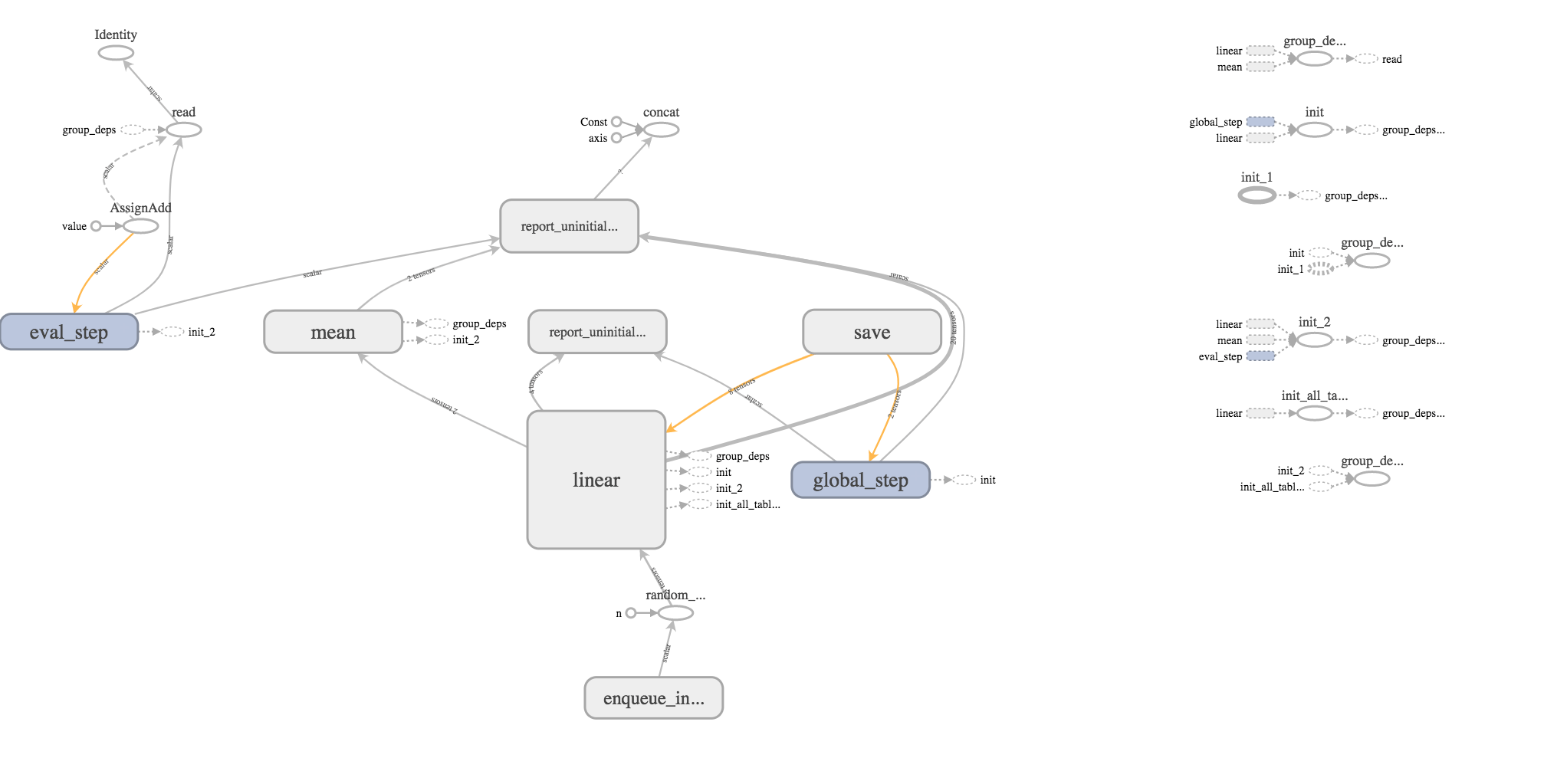Want to share your content on R-bloggers? click here if you have a blog, or here if you don't.

In a recent post, I mentioned three avenues for working with TensorFlow from R:
* The `keras` package, which uses the Keras API for building scaleable, deep learning models * The `tfestimators` package, which wraps Google’s Estimators API for fitting models with pre-built estimators
* The `tensorflow` package, which provides an interface to Google’s low-level TensorFlow API

In this post, Edgar and I use the `linear_classifier()` function, one of six pre-built models currently in the `tfestimators` package, to train a linear classifier using data from the `titanic` package.``````library(tfestimators)
library(tensorflow)
library(tidyverse)
library(titanic)``````

The `titanic_train` data set contains 12 fields of information on 891 passengers from the Titanic. First, we load the data, split it into training and test sets, and have a look at it.

``````titanic_set <- titanic_train %>% filter(!is.na(Age))

# Split the data into training and test data sets
indices <- sample(1:nrow(titanic_set), size = 0.80 * nrow(titanic_set))
train <- titanic_set[indices, ]
test  <- titanic_set[-indices, ]

glimpse(titanic_set)``````
``````## Observations: 714
## Variables: 12
## \$ PassengerId  1, 2, 3, 4, 5, 7, 8, 9, 10, 11, 12, 13, 14, 15, 16...
## \$ Survived     0, 1, 1, 1, 0, 0, 0, 1, 1, 1, 1, 0, 0, 0, 1, 0, 0,...
## \$ Pclass       3, 1, 3, 1, 3, 1, 3, 3, 2, 3, 1, 3, 3, 3, 2, 3, 3,...
## \$ Name         "Braund, Mr. Owen Harris", "Cumings, Mrs. John Bra...
## \$ Sex          "male", "female", "female", "female", "male", "mal...
## \$ Age          22, 38, 26, 35, 35, 54, 2, 27, 14, 4, 58, 20, 39, ...
## \$ SibSp        1, 1, 0, 1, 0, 0, 3, 0, 1, 1, 0, 0, 1, 0, 0, 4, 1,...
## \$ Parch        0, 0, 0, 0, 0, 0, 1, 2, 0, 1, 0, 0, 5, 0, 0, 1, 0,...
## \$ Ticket       "A/5 21171", "PC 17599", "STON/O2. 3101282", "1138...
## \$ Fare         7.2500, 71.2833, 7.9250, 53.1000, 8.0500, 51.8625,...
## \$ Cabin        "", "C85", "", "C123", "", "E46", "", "", "", "G6"...
## \$ Embarked     "S", "C", "S", "S", "S", "S", "S", "S", "C", "S", ...``````

Notice that both `Sex` and `Embarked` are character variables. We would like to make both of these categorical variables for the analysis. We can do this “on the fly” by using the`tfestimators::feature_columns()` function to get the data into the shape expected for an input Tensor. Category levels are set by passing a list to the `vocabulary_list argument`. The Pclass variable is passed as a numeric feature, so no further action is required.

``````cols <- feature_columns(
column_categorical_with_vocabulary_list("Sex", vocabulary_list =
list("male", "female")),
column_categorical_with_vocabulary_list("Embarked", vocabulary_list =
list("S", "C", "Q", "")),
column_numeric("Pclass")
)``````

So far, no real processing has taken place. The data have not yet been evaluated by R or loaded into TensorFlow. Our first interaction with TensorFlow begins when we use the `linear_classifier()` function to build the TensorFlow model object for a linear model.

``model <- linear_classifier(feature_columns = cols)``

Now, we use the `tfestimators::input_fn()` to get the data into TensorFlow and define the model itself. The following helper function sets up the predictive variables and response variable for a model to predict survival from knowing a passenger’s sex, ticket class, and port of embarkation.

``````titanic_input_fn <- function(data) {
input_fn(data,
features = c("Sex", "Pclass", "Embarked"),
response = "Survived")
}``````

`tfestimators::train()` uses the helper function to fit and train the model on the training set constructed above.

``train(model, titanic_input_fn(train))``

The `tensorflow::evaluate()` function evaluates the model’s performance.

``````model_eval <- evaluate(model, titanic_input_fn(test))
glimpse(model_eval)  ``````
``````## Observations: 1
## Variables: 9
## \$ loss                  40.2544
## \$ accuracy_baseline     0.5874126
## \$ global_step           5
## \$ auc                   0.8096247
## \$ `prediction/mean`     0.3557937
## \$ `label/mean`          0.4125874
## \$ average_loss          0.5629987
## \$ auc_precision_recall  0.8102072
## \$ accuracy              0.7132867``````

It’s not a great model, by any means, but an AUC of 0.85 isn’t bad for a first try. We will use R’s familiar `predict()` function to make some predictions with the `test` data set. Notice that this data needs to be wrapped in the `titanic_input_fn()` just like we did for the training data above.

``model_predict <- predict(model, titanic_input_fn(test))``

The following code unpacks the list containing the prediction results.

``````res <- data.frame(matrix(unlist(model_predict[]),ncol=2,byrow=TRUE),
unlist(model_predict[]), unlist(model_predict[]),
unlist(model_predict[]), unlist(model_predict[]))
names(res) <- c("Prob Survive", "Prob Perish",names(model_predict)[2:5])
options(digits=3)
``````##   Prob Survive Prob Perish  logits classes class_ids logistic
## 1        0.380       0.620  0.4899       1         1    0.620
## 2        0.509       0.491 -0.0373       0         0    0.491
## 3        0.380       0.620  0.4899       1         1    0.620
## 4        0.509       0.491 -0.0373       0         0    0.491
## 5        0.781       0.219 -1.2697       0         0    0.219
## 6        0.735       0.265 -1.0180       0         0    0.265``````

Before finishing up, we note that TensorFlow writes quite a bit of information to disk:

``list.files(model\$estimator\$model_dir)``
``````##   "checkpoint"                       "eval"
##   "graph.pbtxt"                      "logs"
##   "model.ckpt-1.data-00000-of-00001" "model.ckpt-1.index"
##   "model.ckpt-1.meta"                "model.ckpt-5.data-00000-of-00001"
##   "model.ckpt-5.index"               "model.ckpt-5.meta"``````

Finally, we use the TensorBoard visualization tool to look at the data flow graph and other aspects of the model.To see all of this, point your browser to address returned by the following command.

``tensorboard(model\$estimator\$model_dir, action="start") ``
``## Started TensorBoard at http://127.0.0.1:5503``